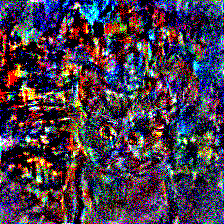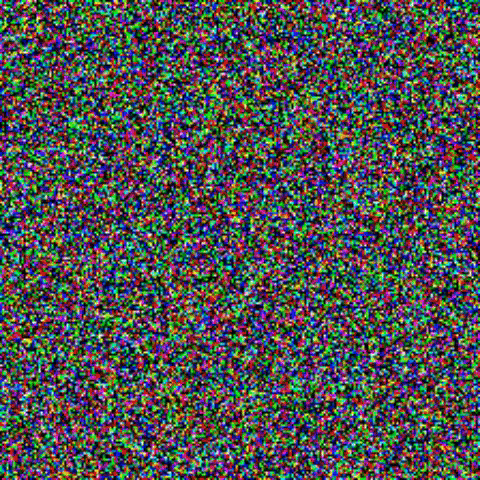# Implementing Neural Style Transfer From Scratch

Hi! I am trying to implement the neural style transfer model from the original Gatys’ paper from scratch. I am aware of the tutorial on the website, but I am trying to implement it myself to see if I understand the model right, also, I am trying to stay as close as possible to the paper.

I have come across some problems, specifically a weird mixture of the content and the style. I can see that something is happening but the results are not pleasing, so I would appreciate any help.

As far as I understand, we need to minimize the sum of the content loss and the style loss. The content loss is the MSE between the activations of the content image and the generated image (noise) from one of the deeper layers of the VGG19 model. The style loss is the MSE between the Gramian matrices (across multiple layers) of style image and the generated image.

I am trying to use Adam optimizer and theoretically it should work as we are performing the gradient descent in the image space.

Here are the images I am trying to merge:

Content and Style images:

Result:Obviously, surely something is not right in my implementation. Here is my code, in case if anyone is able to point me in the right direction.

``````import torch
import torch.nn as nn
import torchvision.models as models
from PIL import Image
from torchvision import transforms
from torch import optim
import torch.nn.functional as F
import numpy as np
import matplotlib.pyplot as plt
import warnings
from torchvision.utils import save_image

warnings.simplefilter('ignore')

def gram(tensor):

def gram_loss(noise_img_gram, style_img_gram, N, M):

cont_img = Image.open('./content_img_1.jpg')
style_img = Image.open('./style_img.jpeg')

# define the transform
transform = transforms.Compose([transforms.Resize((224, 224)),
transforms.ToTensor(),
transforms.Normalize([0.485, 0.456, 0.406],
[0.229, 0.224, 0.225])])

# get the tensor of the image
content_image = transform(cont_img).unsqueeze(0).cuda()
style_image = transform(style_img).unsqueeze(0).cuda()

# define the VGG
class VGG(nn.Module):
def __init__(self):
super(VGG, self).__init__()

# load the vgg model's features
self.vgg = models.vgg19(pretrained=True).features

def get_content_activations(self, x):
return self.vgg[:32](x)

def get_style_activations(self, x):
# block1_conv1, block2_conv1, block3_conv1, block4_conv1, block5_conv1
return [self.vgg[:30](x)] + [self.vgg[:21](x)] + [self.vgg[:12](x)] + [self.vgg[:7](x)] + [self.vgg[:4](x)]

def forward(self, x):
return self.vgg(x)

# init the network
vgg = VGG().cuda().eval()

for param in vgg.parameters():

# get the content activations of the content image and detach them from the graph
content_activations = vgg.get_content_activations(content_image).detach()

# get the style activations of the style image
style_activations = vgg.get_style_activations(style_image)

# unroll the content activations
content_F = content_activations.view(512, -1)

# for every layer in the style activations
for i in range(len(style_activations)):

# unroll the activations and detach them from the graph
style_activations[i] = style_activations[i].squeeze().view(style_activations[i].shape, -1).detach()

# calculate the gram matrices of the style image
gram_matrices = [gram(style_activations[i]) for i in range(len(style_activations))]

# generate the Gaussian noise
noise = torch.randn(1, 3, 224, 224, device='cuda', requires_grad=True)

# pass the noise image pixels to the optimnizer as parameters

# run the iteration
for iteration in range(10000):

# get the content activations of the Gaussian noise
noise_content_activations = vgg.get_content_activations(noise)

# unroll the feature maps of the noise
noise_content_F = noise_content_activations.view(512, -1)

# calculate the loss
content_loss = 1/2. * torch.sum(torch.pow(noise_content_F - content_F, 2))

# get the style activations of the noise image
noise_style_activations = vgg.get_style_activations(noise)

# for every noise style activation layer
for i in range(len(noise_style_activations)):

# unroll the the noise style activations
noise_style_activations[i] = noise_style_activations[i].squeeze().view(noise_style_activations[i].shape, -1)

# calculate the noise gram matrices
noise_gram_matrices = [gram(noise_style_activations[i]) for i in range(len(noise_style_activations))]

# calculate the total style loss
style_loss = 0
for i in range(len(style_activations)):
N, M = noise_style_activations[i].shape, noise_style_activations[i].shape
style_loss += (gram_loss(noise_gram_matrices[i], gram_matrices[i], N, M) / 5.)

style_loss = style_loss.cuda()
total_loss = content_loss + 10000 * style_loss

if iteration % 1000 == 0:
print("Iteration: {}, Content Loss: {}, Style Loss: {}".format(iteration, content_loss.item(), 10000 * style_loss.item()))
save_image(noise, filename='./generated/iter_{}.png'.format(iteration))

total_loss.backward()
``````
4 Likes

Congrats! That doesn’t look all that bad! And I do think that implementing things yourself is one of the best ways to learn.
Now regarding your question: vgg does have “implied” or learned length scales, so maybe your images are too large to have the same length scale?
I think I saw someone experiment with rescaling and style transfer, maybe on the fast.ai forum?

Best regards

Thomas

Hi Tom, I am not sure what you mean by length scales? Do you mean feature map sizes?

Try scaling your two inputs, e.g half the size (well the lengths, so size will be a quarter) before you start.

@alexis-jacq hi, would you mind helping me with this issue by chance?
The style reconstructions have some weird artifacts, like this green pixel tint.1 Like

Tom, you mean decrease the size of the input images?

``````def total_variation_loss(image):
# shift one pixel and get difference (for both x and y direction)
loss = torch.mean(torch.abs(image[:, :, :, :-1] - image[:, :, :, 1:])) + \
torch.mean(torch.abs(image[:, :, :-1, :] - image[:, :, 1:, :]))
return loss
``````